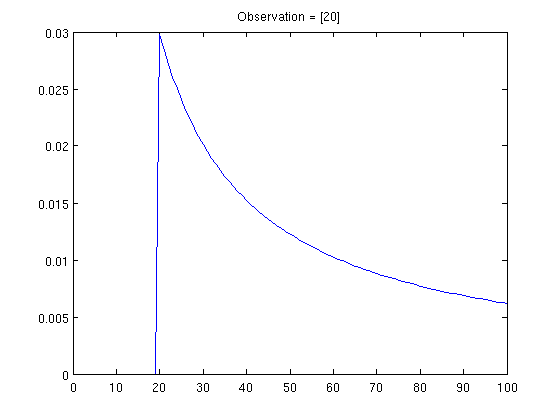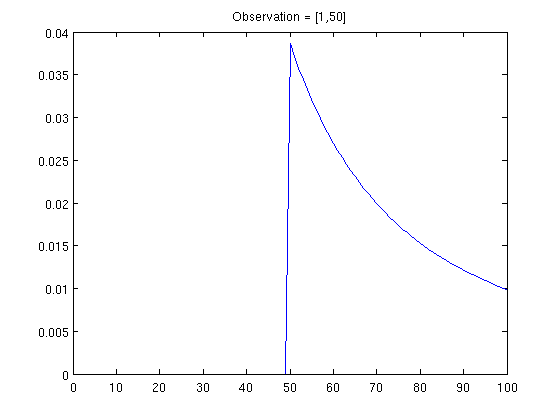## 07 April 2010

### When Maximum Likelihood Doesn't Make Sense

One of the most fun AHA moments in ML or stats is when you see that for multinomial distributions, your naive idea of relative frequency corresponds (through a bunch of calculus) to maximum likelihood estimation. Ditto means of Gaussians, though that's never quite as amazing because Gaussians seems sort of odd beasts anyway. It's nice when our intuitions match what stats tells us.

I'll often watch a DVD, and then leave it in the DVD player until I want to watch something else. Usually it will return to the menu screen and the timer display will go through 0:00, 0:01, 0:02, ..., until it hits the length of the title screen loop, and then go back to 0:00. What I always wonder when I glance up at it is "what is the actual length of the title screen loop?" That is, what is the highest value it'll count up to.

Being a good statistician, I set up a model. First I play the frequentist game. Since I glance up and pretty arbitrary times, my observations can be modeled as a uniform random variable from 0 to some upper bound U, which is the quantity I want to infer.

Supposing that I only make one observation, say 0:27, I want a maximum likelihood estimate of U. It's easy to see that U=27 is the maximum likelihood solution. Anything less than 27 will render my observation impossible. For any U>=27, my observation has probability 1/(U+1), so the ML solution is precisely U=27. Note that even if I observe five observations a <= b <= c <= d <= e, the ML solution is still U=e. Huh. The math works. The model is as correct as I could really imagine it. But this answer doesn't really seem reasonable to me. Somehow I expect a number greater than my observation to come about.

Perhaps I need a prior. That'll solve it. I'll add a prior p(U) and then do maximum a posteriori. Well, it's easy to see that if p(U) is unimodal, and its mode is less than my (highest) observation, then the MAP solution will still be the (highest) observation. If it's mode is more than my (highest) observation, then the MAP solution will be the mode of p(U). If I think about this a bit, it's hard for me to justify a multi-modal p(U), and also hard for me to be happy with a system in which my prior essentially completely determines my solution.

Or I could be fully Bayesian and look at a posterior distribution p(U | data). This will just be a left-truncated version of my prior, again, not very satisfying.

(Note that the "Maximum Likelihood Sets" idea, which I also like quite a bit, doesn't fix this problem either.)

It also really bugs me that only my largest observation really affects the answer. If I get one hundred observations, most of them centered around 0:10, and then one at 0:30, then I'd guess that 0:30 or 0:31 or 0:32 is probably the right answer. But if I observe a bunch of stuff spread roughly uniformly between 0:00 and 0:30, then I'd be more willing to believe something larger.

I don't really have a solution to this dilemma. Perhaps you could argue that my difficulties arise from the use of a Uniform distribution -- namely, that were I to use another distribution, these problems wouldn't really arise. I don't think this is quite true, as described below:

We actually run in to this problem in NLP fairly often. I observe a billion documents and see, in this 1b documents, 100k unique words, many of which are singletons. But I'm not willing to believe that if I see that document 1,000,000,001, that there won't be a new unseen word. Sure it's less likely that I see a new unique word than in document 1001, where it is almost guaranteed, but there's still a relatively large probability that some new word will show up in that next document.

The whole point is that somehow we expect random samples to be representatives of the underlying distribution. We don't expect them to define the underlying distribution. I don't actually expect to ever see the corner cases, unless I've seen everything else.

UPDATE: The Bayesian approach actually does do something reasonable. Here is some Matlab code for computing posteriors with a uniform prior, and results with an upper bound of 100 and various observations are plotted below:Unknown said...

Hi Hal,

This problem is also known as the German Tank Problem (http://en.wikipedia.org/wiki/German_tank_problem).

Cheers,
Brian

Jordan said...

Is it that you find the Bayesian case unsatisfying because of the reset? If the timer stopped at the length of the intro and you only got one peek at the timer (and otherwise had no signals that the intro was still going), then everything would be cool, right?

In which case, you just need to account for the probability of you looking at the readout into your model and rederive your estimate. :)

Ignoring the reset problem, Griffiths and Tenenbaum showed that this sort of problem matches up pretty nicely with human intuition:

http://web.mit.edu/cocosci/Papers/Griffiths-Tenenbaum-PsychSci06.pdf

jg said...

Let's ignore the prior on loop length (say it's flat up to thousands of seconds). If your observation of a single sample from 1..N was 17, then I agree that p(observation) is maximized by guessing N=17. And I also agree that it's nearly certain that the true N is greater than this. I also agree that in the general case p(observation) is maximized by a model that puts N at exactly the maximum sample, ignoring all the other samples.

Suppose you want your estimated N to be too low exactly half the time. Then you need to suppose that your single sample was halfway through the loop. So you'd guess N=33 (because (1+33)/2=17). It looks like for more than one sample, the wikipedia article linked above gives the solution.

Are you absolutely sure that the mode of the posterior p(N|observation) for some arbitrary prior p(N), is the same as the mode of p(N) if it's greater than the observed maximum? That's surprising to me, but even if it's true, it just means that your reasoning needs to integrate over the posterior, since certainly the mean shifts due to the whole observation and not just the maximum.

hal said...

@brian: hah, that's awesome!

@jordan, jonathan: yeah, I may be wrong about the Bayesian analysis case... I think actually all the observations matter. But kind of in a weird way. Obvious the posterior will be zero for any U less than the largest observation. But the smaller observations will have the effect of pulling the posterior lower. So maybe the full Bayesian solution works, too.